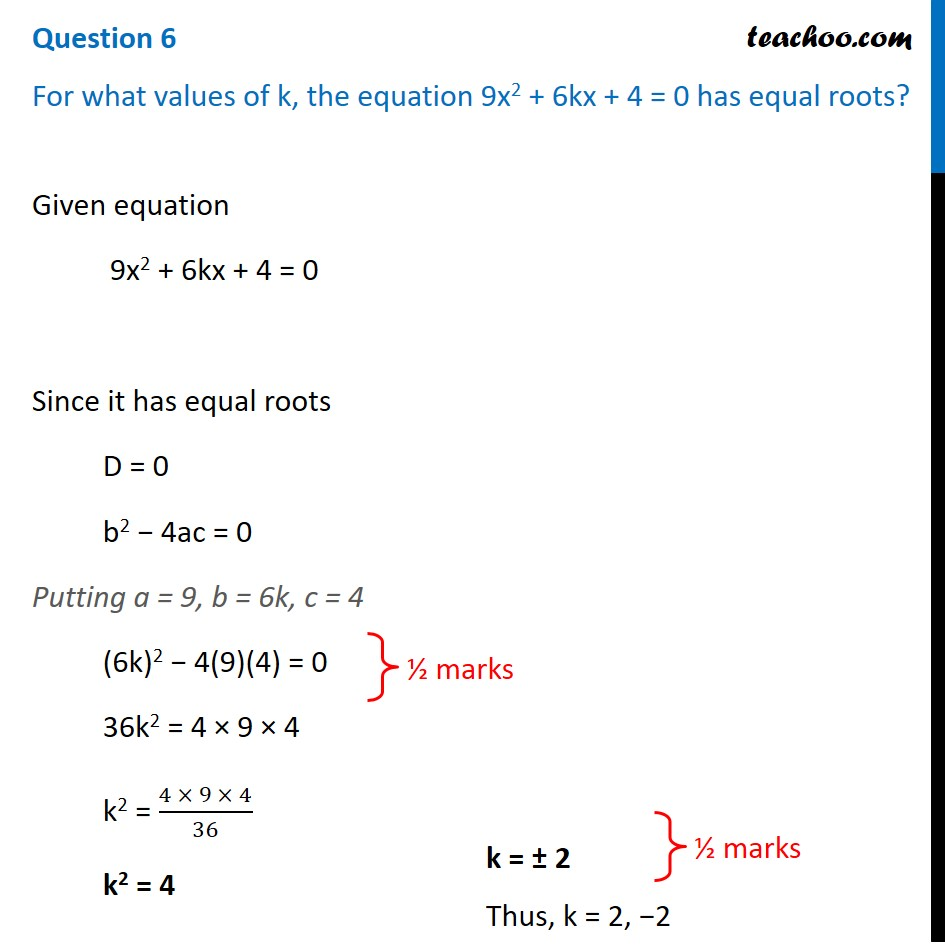## For what values of k, the equation 9x 2 + 6kx + 4 = 0 has equal roots?1. Class 10
2. Solutions of Sample Papers for Class 10 Boards
3. CBSE Class 10 Sample Paper for 2021 Boards - Maths Standard

Transcript

Question 6 For what values of k, the equation 9x2 + 6kx + 4 = 0 has equal roots?Given equation 9x2 + 6kx + 4 = 0 Since it has equal roots D = 0 b2 − 4ac = 0 Putting a = 9, b = 6k, c = 4 (6k)2 − 4(9)(4) = 0 36k2 = 4 × 9 × 4 k2 = (4 × 9 × 4)/36 k2 = 4 k = ± 2 Thus, k = 2, −2